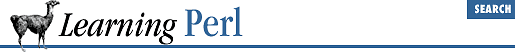home | O'Reilly's CD bookshelfs | FreeBSD | Linux | Cisco | Cisco Exam

### A.6 Chapter 7, Regular Expressions

1. Here are some possible answers:

1. ``` /a+b*/```

2. ``` /\\*\**/``` (Remember that the backslash cancels the meaning of the special character following.)

3. ``` /(\$whatever){3}/``` (You must have the parentheses, or else the multiplier applies only to the last character of ``` \$whatever``` ; this also fails if ``` \$whatever``` has special characters.)

4. ``` /[\000-\377]{5}/``` or ``` /(.|\n){5}/``` (You can't use dot alone here, because dot doesn't match newline.)

5. ``` /(^|\s)(\S+)(\s+\2)+(\s|\$)/``` (``` \S``` is nonwhitespace, and ``` \2``` is a reference to whatever the "word" is; the caret or whitespace alternative ensures that the ``` \S+``` begins at a whitespace boundary.)

1. One way to do this is:

```while (<STDIN>) {
if (/a/i && /e/i && /i/i && /o/i && /u/i) {
print;
}
}```

Here, we have an expression consisting of five match operators. These operators are all looking at the contents of the ``` \$_``` variable, which is where the control expression of the ``` while``` loop is putting each line. The match operator expression will be true only when all five vowels are found.

Note that as soon as any of the five vowels are not found, the remainder of the expression is skipped, because the ``` &&``` operator doesn't evaluate its right argument if the left argument is false.

2. Another way to do this is:

```while (<STDIN>) {
if (/a.*e.*i.*o.*u/i) {
print;
}
}```

This answer turns out to be easier than the other part of this exercise. Here we have a simple regular expression that looks for the five vowels in sequence, separated by any number of characters.

3. One way to do this is:

```while (<>) {
print if
(/^[^aeiou]*a[^eiou]*e[^aiou]*i[^aeou]*o[^aeiu]*u[^aeio]*\$ );
}```

Ugly, but it works. To construct this, just think "What can go between the beginning of the line, and the first a?," and then "What can go between the first a and the first e?" Eventually, it all works itself out, with a little assistance from you.

2. One way to do this is:

```

while (<STDIN>) {
chomp;
(\$user, \$gcos) = (split /:/)[0,4];
(\$real) = split(/,/, \$gcos);
print "\$user is \$real\n";
}```

The outer ``` while``` loop reads one line at a time from the password-format file into the ``` \$_``` variable, terminating when there are no more lines to be read.

The second line of the ``` while``` loop body breaks the line apart by colons, saving two of the seven values into individual scalar variables with hopefully meaningful names.

The GCOS field (the fifth field) is then split apart by commas, with the resulting list assigned to a single scalar variable enclosed in parentheses. The parentheses are important: they make this assignment an array assignment rather than a scalar assignment. The scalar variable ``` \$real``` gets the first element of the list, and the remaining elements are discarded.

The ``` print``` statement then displays the results.

3. One way to do this is:

```while (<STDIN>) {
chomp;
(\$gcos) = (split /:/);
(\$real) = split(/,/, \$gcos);
(\$first) = split(/\s+/, \$real);
\$seen{\$first}++;
}
foreach (keys %seen) {
if (\$seen{\$_} > 1) {
print "\$_ was seen \$seen{\$_} times\n";
}
}```

The ``` while``` loop works a lot like the ``` while``` loop from the previous exercise. In addition to splitting the line apart into fields and the GCOS field apart into the real name (and other parts), this loop also splits apart the real name into a first name (and the rest). Once the first name is known, a hash element in ``` %seen``` is incremented, noting that we've seen a particular first name. Note that this loop doesn't do any ``` print``` 'ing.

The ``` foreach``` loop steps through all of the keys of ``` %seen``` (the first names from the password file), assigning each one to ``` \$_``` in turn. If the value stored in ``` %seen``` at a given key is greater than 1, we've seen the first name more than once. The ``` if``` statement tests for this, and prints a message if so.

4. One way to do this is:

```while (<STDIN>) {
chomp;
(\$user, \$gcos) = (split /:/)[0,4];
(\$real) = split /,/, \$gcos;
(\$first) = split /\s+/, \$real;
\$names{\$first} .= " \$user";
}
foreach (keys %names) {
\$this = \$names{\$_};
if (\$this =~ /. /) {
print "\$_ is used by:\$this\n";
}
}```

This program is like the previous exercise answer, but instead of merely keeping a count, we append the login name of the user to the ``` %names``` element that has a key of the first name. Thus, for Fred Rogers (login ``` mrrogers``` ), ``` \$names{"Fred"}``` becomes ``` "``` ``` mrrogers"``` , and when Fred Flintstone (login ``` fred``` ) comes along, we get ``` \$names{"Fred"}``` as ``` "``` ``` mrrogers``` ``` fred"``` . After the loop is complete, we have a mapping of all of the first names to all of the users that have them.

The ``` foreach``` loop, like the previous exercise answer, then steps through the resulting hash. However, rather than testing a hash element value for a number greater than one, we must see now if there is more than one login name in the value. We do this by saving the value into a scalar variable ``` \$this``` and then seeing if the value has a space after any character. If so, the first name is shared, and the resulting message tells which logins share that first name.A.5 Chapter 6, Basic I/OA.7 Chapter 8, Functions